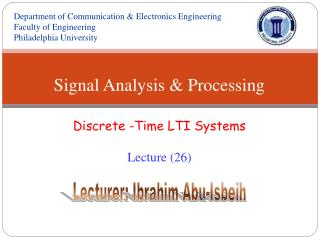Download PresentationSignal Analysis & Processing Discrete -Time LTI Systems Lecture (26)

# Signal Analysis & Processing Discrete -Time LTI Systems Lecture (26) - PowerPoint PPT Presentation

Department of Communication & Electronics Engineering Faculty of Engineering Philadelphia University. Signal Analysis & Processing Discrete -Time LTI Systems Lecture (26). Lecturer: Ibrahim Abu-Isbeih. h [ n ]. d [ n ]. LTI System. Figure 3.20. 3.6 Discrete-Time LTI Systems:.I am the owner, or an agent authorized to act on behalf of the owner, of the copyrighted work described.
Download Presentation## Signal Analysis & Processing Discrete -Time LTI Systems Lecture (26)

Download Policy: Content on the Website is provided to you AS IS for your information and personal use and may not be sold / licensed / shared on other websites without getting consent from its author.While downloading, if for some reason you are not able to download a presentation, the publisher may have deleted the file from their server.

- - - - - - - - - - - - - - - - - - - - - - - - - - E N D - - - - - - - - - - - - - - - - - - - - - - - - - -
Presentation Transcript
1. Department of Communication & Electronics Engineering Faculty of Engineering Philadelphia University Signal Analysis & ProcessingDiscrete -Time LTI SystemsLecture (26) Lecturer: Ibrahim Abu-Isbeih

2. h[n] d[n] LTI System Figure 3.20 3.6 Discrete-Time LTI Systems: In this section, we develop the fundamental input/output relationship for discrete-time, linear, time-invariant (LTI) systems. It is useful to characterize an LTI system in terms of its impulse response. The impulse response of an LTI system is defined as the output of the LTI system due to a unit impulse signal input applied at time t=0 as illustrated in Figure 3.20. i.e., if x[n]=d [n] then y[n]=h[n]. Signal Analysis & Processing

3. y[n] x[n] LTI System Figure 3.21 The impulse response of the LTI system shown in Figure 3.20 is denoted by h[n], whereh[n] is the response tod[n]. Consider a discrete-time LTI system with input x[n] shown in Figure 3.21, if the impulse response of the LTI system is h[n] then the output of this LTI system is given by: Signal Analysis & Processing

4. Hence, the impulse response of the LTI system h[n] is the only function needed to characterize the system completely with respect to its input and output. The output of an LTI system with impulse response h[n] is then given by the convolution sum: Utilizing the commutivity property of convolution, we can also write the above equation as: Signal Analysis & Processing

5. The procedure of evaluating the convolution sum (the LTI system output) was discussed in session two. For example, the output of a discrete-time LTI system with impulse response h[n]=u[n] to the input x[n]=3u[n-2] is given by: Signal Analysis & Processing

6. x[n] h[n] 3 2 1 1 1 n n -1 3 0 2 1 -1 3 0 2 1 Example 3.6.1:Find the output of a discrete-time LTI system with impulse response h[n]=u[n]-u[n-2] to the input Solution:The output of the system is given by: To find the above convolution sum: - Graph x[n] and h[n]: * Signal Analysis & Processing

7. x[k] h[k] 3 2 1 1 1 k k -1 3 0 2 1 -1 3 0 2 1 • Replace n with kin x[n] and h[n] • Graph h[n-k] h[n-k] 1 1 1 k n-1 n n-2 1 0 Signal Analysis & Processing

8. x[k] 3 2 k -1 3 0 2 1 According to the convolution sumconvolution can be divided into the following cases: • 1. For n< 0: The functions x[k] and h[n-k] • do not overlap, then y[n]=0 h[n-k] 1 1 1 k n-1 n n-2 1 0 Signal Analysis & Processing

9. x[k] 3 2 k -1 3 0 2 1 • 2. For n =0: h[n-k] 1 1 1 k -2 1 -1 0 Signal Analysis & Processing

10. x[k] 3 2 k -1 3 0 2 1 • 3. For n =1: h[n-k] 1 1 1 k -1 1 0 Signal Analysis & Processing

11. x[k] 3 2 k -1 3 0 2 1 • 4. For n =2: h[n-k] 1 1 1 k 0 2 1 Signal Analysis & Processing

12. x[k] 3 2 k -1 3 0 2 1 • 5. For n =3: h[n-k] 1 1 1 k 0 1 2 3 Signal Analysis & Processing

13. x[k] 3 2 k -1 3 0 2 1 • 6. For n ≥ 4: h[n-k] 1 1 1 k 0 1 n-1 n n-2 2 Signal Analysis & Processing

14. Then the output of the system is : y[n] 5 5 3 2 n -1 4 0 1 3 2 Signal Analysis & Processing

15. s[n] u[n] LTI System Figure 3.22 Step-Response: In this lecture we introduce the step-responseof discrete-time LTI systems. The step-response of an LTI system with impulse response h[n] is defined as the output of the LTI system due to the unit step signal input as illustrated in Figure 3.22. i.e., if x[n]=u[n] then y[n]=s[n]=u[n]*h[n]. Signal Analysis & Processing

16. h[n] 5 5 5 5 ……… 2 2 2 2 n 0 1 2 4 -1 3 5 6 Example 3.6.2: Find the step-response of an LTI system with impulse response h[n]=2u[n]+3u[n-4]. Signal Analysis & Processing

17. Solution: To find the step-response of the given system, let x[n]=u[n] then the step-response is: Signal Analysis & Processing

18. h[n] 3 1 n 4 -2 -1 3 0 2 1 -1 Example 3.6.3:Find the step-response of an LTI system with impulse response Signal Analysis & Processing

19. Solution:The step-response of the system is: wherex[n]=u[n]. Using the convolution properties we have: Signal Analysis & Processing